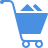Cart (0)

It is a procedure for selecting independent variables for a model from a large number of available variables. It is done two ways: forward estimation and backward estimation. In the forward estimation, one independent variable is added at a time to the regression equation on the basis of its partial correlation coefficient. That is, first include the variable with the highest partial correlation coefficient, then the one with the second highest partial coefficient, and so on. After each variable is added, the partial F-test on the last entered variable is performed. The process is stopped as soon as the partial F-test indicates that the last entered variable is insignificant. In the backward estimation, all the independents are included in the equation. Then the insignificant ones are dropped from the model, one at a time.

Previous Entry
Next Entry